# Data Augmentation by fastai v1

Source: Deep Learning on Medium

### Abstract

In order to improve the performance of ConvNet-type Deep Learning networks by increasing the number of training images, this article presents the 4 most widely used solutions in the academic and industrial world: the collection of more images, the Transfer Learning, the use of Data Augmentation (DA) techniques and the generation of new images via GANs. In particular, this article presents in detail the Data Augmentation, its interest for purposes of generalization, its techniques and its conditions of practice. In order to allow the reader to reproduce the results presented in this article, the code implemented via the library fastai v1 is given in a Jupyter Notebook. This library makes it possible to easily apply all Data Augmentation techniques in order to multiply training images and thus improve the performance of Deep Learning networks. Moreover, I recall at the beginning of the article what Artificial Intelligence is today in order to contextualize the interest of knowing Data Augmentation techniques and I also underline the importance of choosing training images according to the context of use and the objective of the network to train. Finally, I insist that the training images (real, transformed or generated) must be the same as those used by a human to achieve the same goal.

### AI Explained

#### Definition

Artificial Intelligence (AI) ) can have several interpretations but in a general way, the AI ​​has the purpose of reproducing an action performed by animals or humans (note: with the discovery of generative models such as in particular Generative Adversarial Networks or GANs in 2014, the AI ​​can now surpass this process of reproduction by being able to create new possibilities like images appearing to be real as described in “Progressive Growing of GANs for Improved Quality, Stability, and Variation” in February 2018).

#### Deep Learning

Perhaps we will someday create “biological” AI, but until now, AI is created by means of a computer program called an algorithm whose functioning mimics the cognitive processes, ie the processes engaged by the brain to acquire knowledge and decide on an action. The most used architectures today are Neural Networks or DeepDeep Learning.

#### Learning

It is not a question of coding a set of rules defined in advance. On the contrary, it is for the algorithm to learn rules by interaction with the environment as an animal or a human would do (ie, from training data).

For example, the creation of a visual classifier that can distinguish different objects will be done by feeding the image algorithm of these objects and not by coding a set of rules defined by specialists and supposed to perfectly describe each of the objects. The current state of the art is to use a ConvNet (Convolutional Neural Network) for this type of task.

#### Training Data

This imitation of the animal or man in the learning procedure means that you must provide the same training data to the algorithm as you will study yourself.

Keeping the same example of the visual classifier, it will be necessary to ensure compliance with this rule when acquiring the image database that will be used to drive the algorithm and when a series of transformations called Data Augmentation will be applied in order to multiply their number and increase the variance of representation of their main characteristics.

Note: the Deep Learning research on techniques to improve the performance of ConvNet in particular is interested since 2017 in the creation of mixed images for their training, images that do not look like real life images but that give surprising or even better results than conventional Data Augmentation techniques (crop, horizontal flip and random brightness / contrast changes). However, as indicated in the research paper “Improved Mixed-Example Data Augmentation” of October 2018, the scientists can not for the moment explain the reasons, we will remain in this article on the line commonly accepted that Deep Learning networks must be trained with the same type of data that would be used by a human to perform the same task.

### Learning to generalize

In order to be able to be used on new data, a Deep Learning algorithm must not have been specialized during its training (over-fitting). On the contrary, he must have learned to generalize.

#### Data and Data Augmentation

This means that his training must have allowed him to learn the main features of a dataset.

For this, it is necessary that:

1. (data space) the space of the learning data covers the spectrum of possibilities, ie it contains the greatest number of different examples corresponding to the context of use of the algorithm,
2. (features space) The feature space of the learning data also covers the spectrum of possibilities, ie it contains the largest possible number of representations of each characteristic of the data.

To satisfy the first point, we must therefore collect the largest possible variety of training images corresponding to the context of use and the objective of our algorithm (see paragraph “Images!”). Techniques for generating images using GANs for example can also be used (see section “Generating images”).

And to satisfy the second point, we have to apply Data Augmentation techniques to the training images at our disposal, the most common ones being affine transformations (resizing, random crop (random part of an image), horizontal and / or vertical flip, then rotation, brightness and contrast variation, wrap (perspective)). There are also non-affine transformations such as jitter (random noise) and cutout (random black squares).

Note: the dropout can also be considered as a DA (adding noise) technique but as it applies in the hidden layers of the network and not directly on the input images of the network, we do not consider it as such in this article.

Let’s go back to our example of visual classifier via a ConvNet to better understand these 2 points. During his training, the first layers of a ConvNet will learn to detect basic geometrical shapes such as a straight line for example invariant in translation and in rotation, while the last layers will be able to detect more complex shapes by combining information sent by the previous layers. The performance of the ConvNet is therefore largely based on that of the first layers, which can be properly trained themselves only if the training images present:

• (first point) a sufficient variety of possibilities (for example, any type of mango, all sizes, colors and in all possible situations from the tree to the plate if the objective is to lead a visual classifier to recognize a mango in all situations),
• (second point: Data Augmentation ) and that all the variants of the main characteristics are also present in the training images (in our example, this implies that a mango must be presented in all the orientations, according to all the points of view and according to different luminosities and contrasts, and not just aside for example).

Let’s take another example to understand our second point. If for example the objects to be detected in the images are straight lines and all the lines are all in the same position (say vertical), with the same size, the same brightness and contrast, the ConvNet can not learn that a straight line may have several orientations (rotation), several sizes (length, width and thickness), several brightnesses and contrasts (different conditions of taking pictures and different qualities of the cameras). Thus, if we do not apply DA transformations to these images on the right (see figure below) in order to diversify the variants of what is a straight line, the ConvNet will not be able to learn it and its performance will be bad when it will be used with new images presenting different situations because it will have been specialized on training images (over-fitting).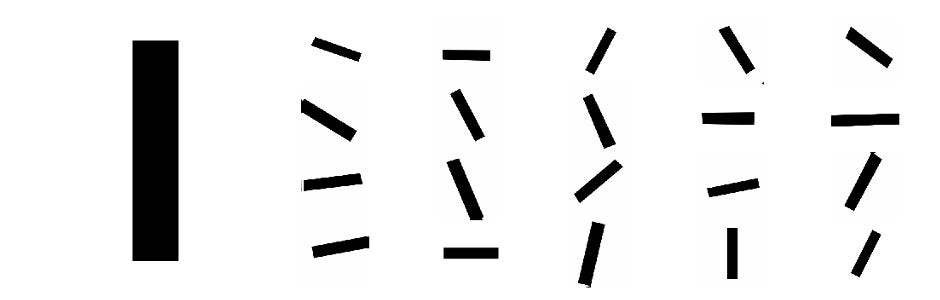On the left, a vertical line. On the right, the same image after some transformations by Data Augmentation.

Thus, if having a large number of training images is (often) a necessary condition to obtain a powerful algorithm of Deep Learning, apply appropriate techniques (it is not always appropriate to apply a vertical flip) of Data Augmentation in order to present to the algorithm the greatest possible number of variants of the main characteristics that it must learn is a MANDATORY condition.

#### Images!

Let us return in this paragraph to the first point mentioned above: the number of training data applied to the case of images.

2012 | 1000 images per category

If the algorithms of Deep Learning began to produce disruptive results from 2012 in the visual recognition (man, car, cat, flower …), it was thanks to the mass use of labeled images .

Indeed, if the network of artificial neurons AlexNet, winner of the 2012 edition of the competition ImageNet, managed to reduce the misclassification of images from 26.2% to 15.3%, it’s because its creators (in particular, Alex Krizhevsky and Geoffrey Hinton) had 1.2 million labeled images belonging to 1000 categories to train it.

Without this significant amount of images and also the use — already — of Data Augmentation techniques such as random scaling, crop and flip horizontal as well as RGB color intensity changes, the AlexNet network, its 7 layers and its 60 million parameters would not have reached this level of performance despite its 6 days of training (…).

2019 | 100 images by category

And in 2019? Is it still necessary to have a large number of images (and 6 days of training …) to train a Deep Learning network to achieve performance around 90% to 95% in visual recognition?

The answer is no (note: to get a better performance close to 100%, however, you will need both much more images, a deeper architecture of neural network type ResNet 101 and more computing capabilities via multiple GPUs in parallel as demonstrated by the Google search published in July 2017 “Revisiting Unreasonable Effectiveness of Data in Deep Learning Era”: the performance of a ConvNet is algorithmically related to the number of training images).

Why? The main reason is transfer learning, ie the reuse of already trained algorithms. Indeed, each winning network of the ImageNet competition has so far put online the values ​​of its parameters after training. A person wishing to develop a visual classifier, for example, does not start from scratch. It will specialize with a few tens or hundreds of images a network already trained (fine-tuning).

Finally, in order to multiply its training images and increase the presentation variance of the main characteristics of the objects to be detected (see the “Data Augmentation explained” paragraph), it will use techniques Data Augmentation.

Thus, thanks to Transfer Learning and Data Augmentation techniques, it is no longer useful to have thousands of training images per category. A hundred images per category are enough (or even less if the categories are very different visually).

#### Generating Images

After collecting more images and using Data Augmentation techniques, a third solution exists to get more training images: Generative Adversarial Networks (GAN) that can generate new images.

Invented in 2014 by Ian GoodFellow, the GANs have been used in numerous applications of text authoring (VGANs) or images (DCGANs). They can also transfer the style from one image to another (CycleGAN), creating a new image. These generated images could be used for example to drive a car to drive at night or in the rain using only data collected on sunny days. In the case of location recognition, we could similarly generate night images from daytime images for training purposes of our algorithm.

It has been shown that such use of GANs, even with relatively small datasets, is effective in driving a Deep Learning Network (“DeLiGAN: Generative Adversarial Networks for Diverse and Limited Data” in June 2017). As a result, they have been extremely effective in augmenting data sets such as the use of CycleGANs presented in this other project “ The Effectiveness of Data Increase in Image Classification using Deep Learning” in December 2017.

#### Watch out for the “selection” of images!

One more thing to do before seeing the Data Augmentation technique code: training images must match the context of use and the purpose of the visual network to be trained (for example, if it is a fruit classifier in the supermarket, it is not useful to download images of fruit hanging from the trees) but conversely, do not over-restrict / select the type of images (for example, for a classifier of fruits in the supermarket, it is necessary to keep also the images of the stalls for example and not to keep only the images with the fruit in close-up).

This is the nature and strength of a visual neural network: if the training images covers a wide range of possibilities, it will learn to recognize the object in question in multiple situations and will be then very effective in use mode. It will not be necessary to restrict a future user of this network in the type of images to present to him to obtain a prediction.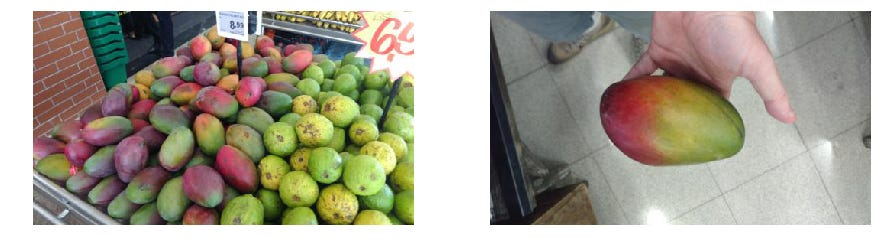Keep these 2 images to train your visual mango classifier! Your Deep Learning network will be more powerful when it has been trained with images covering a wide range of possibilities.

#### Not any Data Augmentation technique!

Let’s go back to this paragraph on the second point mentioned above: Data Augmentation techniques and their right use.

Example 1: classify according to the language of books image

If I ask you to learn to classify images of books by reading the language used on the front page, it is natural to provide images in the following visual form:

But for the training of our AI algorithm whose objective is also to classify images of books according to language, do the following transformations seem to you to be useful for multiplying training images?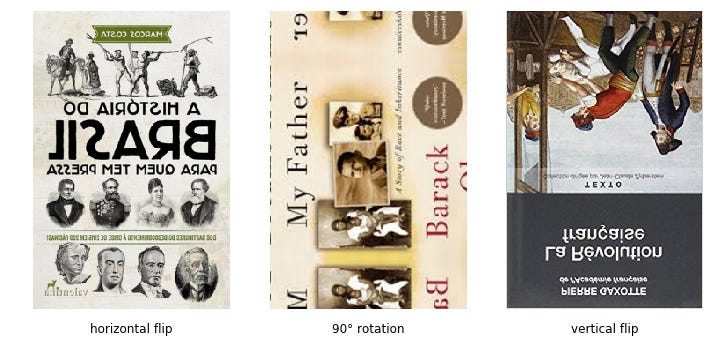Pages of book covers after transformations (horizontal flip, 90 ° rotation, vertical flip).

No. As a human, these transformations would only create images that confuse your learning. It’s the same for our AI algorithm.

Example 2: categorizing fruit images

If I now ask you to learn how to classify fruit images, it is natural to provide you with images in the following visual form:

And if we apply the same transformations to these fruit images as those previously applied to the book images, what will be your opinion about their relevance in the training of our AI algorithm whose goal is now to classify fruit images?

No problem. An apple, a mango or an orange keeps exactly the same visual characteristics after horizontal / vertical flip or rotation transformations. These transformations, which were not relevant in the previous case, are relevant here as much for a human as for an AI algorithm.

### (Easy) Data Augmentation with fastai v1

As the AlexNet team showed us in 2012, the world did not wait for the fastai v1 library released in October 2018 to be able to make the Data Augmentation on the images but what marks a rupture, it is the usability of the tools fastai for the creation of images potentially in infinite number: only 2 lines of code ! (1 line of definition of the transformations and 1 line to apply these transformations to the images). It should be noted that we can modulate the application of these transformations by a probability p (cf. Randomness in fastai v1).

Take the example of the photo below of the Eiffel Tower in Paris and look at the possibilities of transformation by fastai v1.

#### Code

In the rest of this article and in the associated notebook, we will use the function `get_img()` in order to access the images , `plots_of_one_image()` in order to display them after transformations by the function `apply_tfms()` of fastai v1 (credit: the codes used below and in the notebook are derived or inspired by those of “Images transforms” of fastai v1).

After having installed fastai v1, here is the code to insert at the beginning of your notebook:

`# No need to reload the notebook if any change in the fastai library%reload_ext autoreload%autoreload 2`
`# Display images in the notebook%matplotlib inline`
`# Import the vision library from fastai from fastai.vision import *`
`# Function that returns an image from its urldef get_img(img_url): return open_image(img_url)`
`# Function that displays many transformations of an imagedef plots_of_one_image(img_url, tfms, rows=1, cols=3, width=15, height=5, **kwargs):    img = get_img(img_url)    [img.apply_tfms(tfms, **kwargs).show(ax=ax)         for i,ax in enumerate(plt.subplots(                               rows,cols,                               figsize=(width,height).flatten())]`

#### Transformations by default ( get_transforms)

[ `get_transforms()` in fastai v1 ]

Fastai v1 has a method `get_transforms()` which applies default and random transformations with a probability of 75%: crop, horizontal flip, zoom up to 1.1, brightness and contrast, wrap (perspective). It returns 2 sets of transformations, one for the training images (`get_transforms()`), and the other for the validation ones (`get_transforms()`).

`tfms = get_transforms()plots_of_one_image(img_url,tfms)`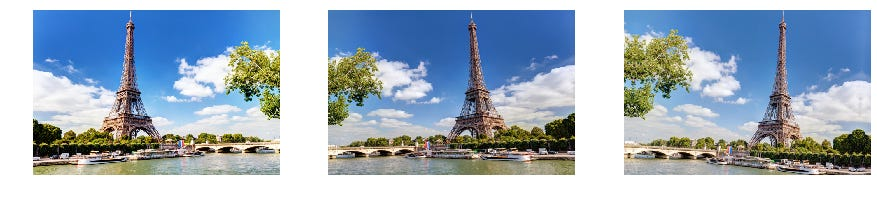3 photos of the Eiffel Tower generated by the use of get_transforms ()

#### Changing the size (size)

[ `size` in fastai v1 ]

Training images have rarely an identical size. It is possible by using the argument `size` in the function `apply_tfms()` to generate images of the same size, square or rectangle, which will thus benefit from the computing power of the GPU during the training of the neural network.

`tfms = get_transforms()plots_of_one_image(img_url,tfms,size=224)`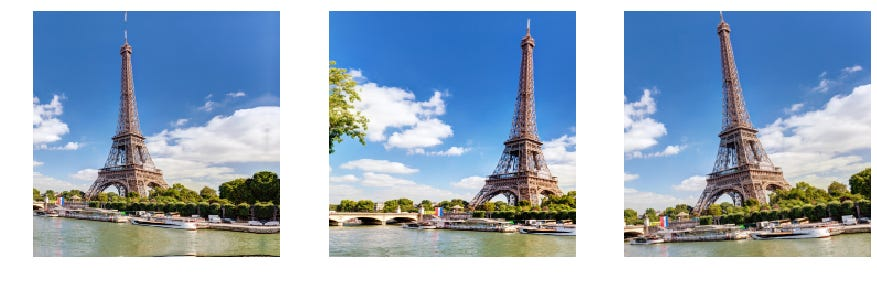3 photos of the Eiffel Tower generated by using get_transforms () + size = 224

#### Translation (rand_crop)

[ `rand_crop()` in fastai v1 ]

Fastai v1 has a function `rand_crop()`that randomly applies crops (with a probability of p) for a size defined by the argument `size` in the function `apply_tfms()`. The result is like applying random translations to the image.

Note: `rand_crop()` is the minimal default transformation that is applied by fastai v1 to the batches of the train dataset. All other default transformations for training images are contained in `get_transforms()`.

`tfms = [rand_crop(p=1.)]plots_of_one_image(img_url,tfms,size=224)`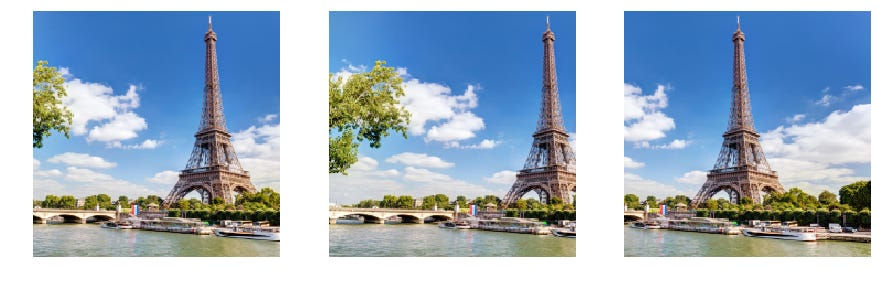3 photos of the Eiffel Tower generated by the use of [rand_crop (p = probability)]

[ `crop_pad()` in fastai v1 ]

Fastai v1 has a function `crop_pad()` that centrally crops for a size defined by the argument `size` in the function `apply_tfms()`.

Note: `crop_pad()` (returned by (`get_transforms()`) is the default transformation that is applied by fastai v1 to batches in the validation dataset.

`tfms = [crop_pad()]get_img(img_url).apply_tfms(tfms,size=224).show(figsize=(15,5))`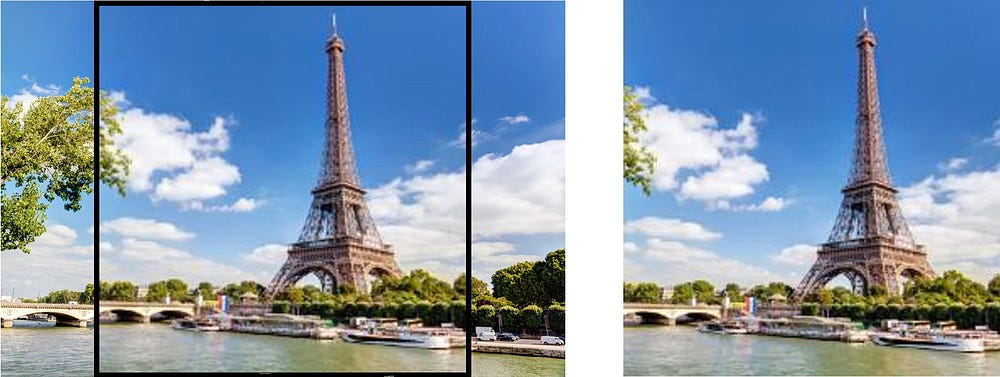The image on the right is extracted from the image on the left after a resize and then a crop centered with [crop_pad ()]

#### Complete the missing pixels (padding_mode)

[ `padding_mode` in fastai v1 ]

When rotating an image for example, it can have missing pixels (black pixels in photo below): the argument `padding_mode='reflection'` (default) or `padding_mode='border'` in the function `apply_tfms()` allow to complete them.

`tfms = get_transforms()plots_of_one_image(img_url,tfms,size=224,padding_mode='reflection')`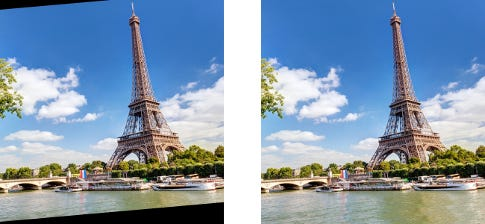When rotating an image, it may have missing pixels (black pixels): padding_mode = “reflection” (default) or padding_mode = “border” allows to complete them.

#### Rotate

[ `rotate()` dans fastai v1 ]

Fastai v1 has a function `rotate()` that rotates randomly (with probability of p) an angle between degree_min and degree_max (if degree_max = degree_min, the rotation angle is always the same).

`tfms = [rotate(degrees=(-30,30), p=1.0)]plots_of_one_image(img_url,tfms)`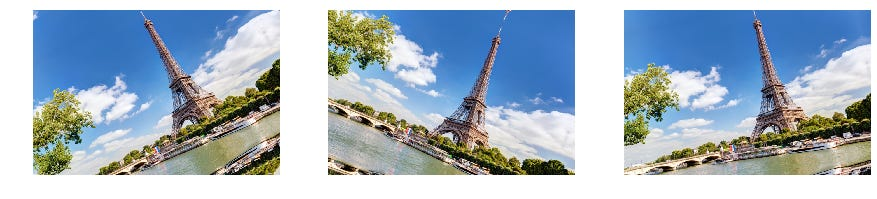3 photos of the Eiffel Tower generated by using [rotate (degrees = (min_degree, max_degree), p = probability)]

#### Brightness

[ `brightness()` dans fastai v1 ]

Fastai v1 has a function `brightness()` that applies randomly brightness changes (with a probability of p) between change_min and change_max (change = 0 transforms inblack image, change = 1 in white and change = 0.5 does not apply transformation). In a way, this transformation (like that of contrast) can simulate the difference in quality between digital cameras like smartphones.

`tfms = [brightness(change=(0.1, 0.9), p=1.0)]plots_of_one_image(img_url,tfms)`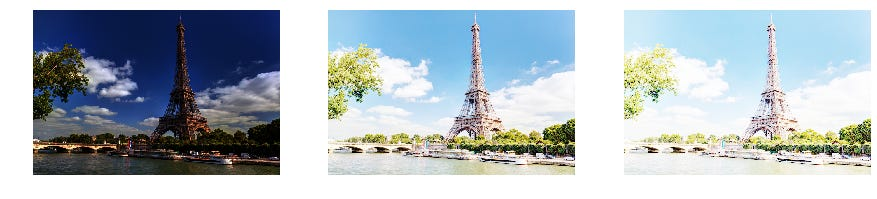3 photos of the Eiffel Tower generated by using [brightness (change = change_min, change_max), p = probability )]

#### Contrast (contrast)

[ `contrast()` in fastai v1 ]

Fastai v1 has a function `contrast()` that applies randomly contrast changes (with a probability of p) between scale_min and scale_max (scale = 0 transforms the image in gray, scale> 1 in very contrasted image and scale = 1 does not apply transformation). In a way, this transformation (like that of brightness) can simulate the difference in quality between digital cameras like smartphones.

`tfms = [contrast(scale=(0.5, 2.), p=1.)]plots_of_one_image(img_url,tfms)`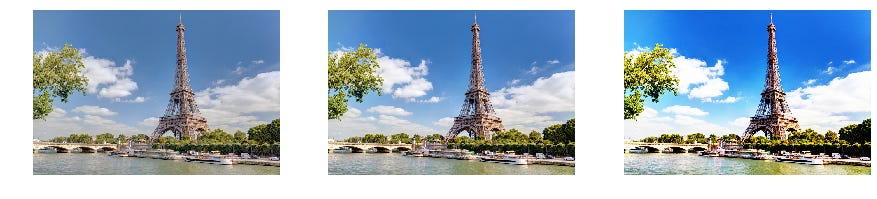3 photos of the Eiffel Tower generated by using [contrast (scale = (scale_min, scale_max), p = probability)]

#### Noise (jitter)

[ `jitter()` in fastai v1 ]

Fastai v1 has a function `jitter()` that introduces randomly noise of a certain level of magnitude (with a probability of p).

`fig, axs = plt.subplots(1,3,figsize=(20,5))for magnitude, ax in zip(np.linspace(-0.05,0.05,5), axs):    tfms = [jitter(magnitude=magnitude, p=1.)]    get_img(img_url).apply_tfms(tfms)                    .show(ax=ax,title=f’magnitude={magnitude:.2f}’)`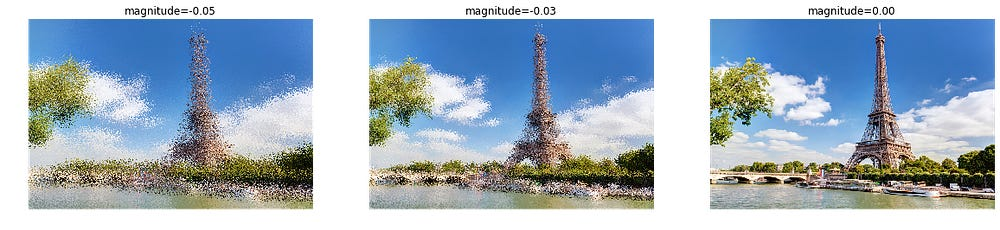3 photos of the Eiffel Tower generated by the use of [ jitter (magnitude = magnitude, p = probability)]

#### Perspective (symmetric_wrap)

[ `symmetric_wrap()` dans fastai v1 ]

Fastai v1 has a function `symmetric_wrap()` that randomly introduces perspective (with a probability of p).

`tfms = [symmetric_warp(magnitude=(-0.2,0.2), p=1.)]plots_of_one_image(img_url,tfms,padding_mode='zeros')`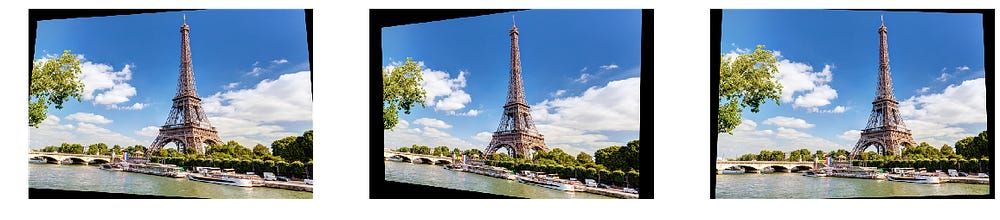3 photos of the Eiffel Tower generated by the use of [symmetric_wrap (magnitude = ( mag_min, mag_max), p = probability)]

#### Zoom (zoom)

[ `zoom()` in fastai v1 ]

Fastai v1 has a function `zoom()` which allows to zoom randomly (with a probability of p).

`fig, axs = plt.subplots(1,3,figsize=(20,5))for scale, ax in zip(np.linspace(1.,2.5,3), axs):    tfms = [zoom(scale=scale, p=1.)]    get_img(img_url).apply_tfms(tfms)                    .show(ax=ax,title=f'scale={scale:.2f}')`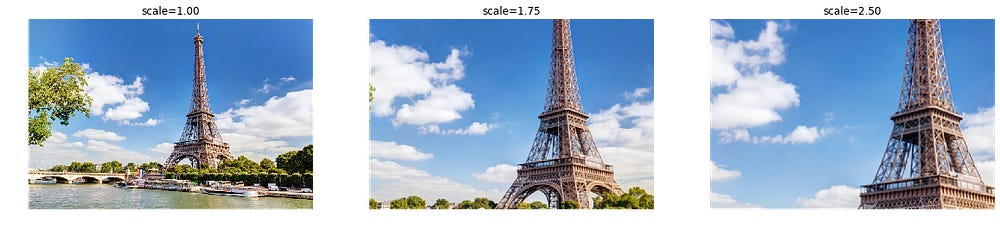3 photos of the Eiffel Tower generated by the use of [ zoom (scale = scale, p = probability)]

#### Cutout (cutout)

[ `cutout()` in fastai v1 ]

A function`cutout()` is used to randomly display (with a probability of p) black squares in an image (number and size between min and max), forcing the ConvNet network to consider the context and not just learning to recognize features in isolation (read “Improved Regularization of Convolutional Neural Networks with Cutout”, November 2017). It allows to train a neural network with missing information, forcing it therefore to generalize.

`tfms = [cutout(n_holes=(1,4), length=(10, 160), p=1.)]plots_of_one_image(img_url,tfms)`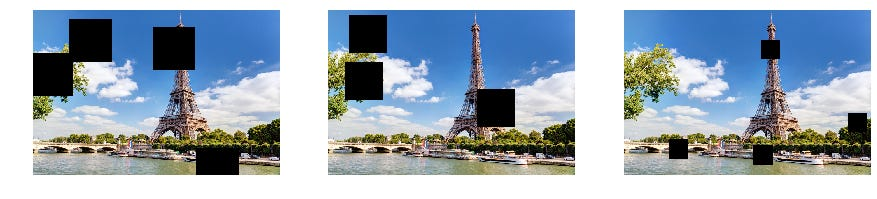3 photos of the Eiffel Tower generated with a cutout transformation [cutout(n_holes=(h_min,h_max), length=(l_min,l_max))]

### Using the DA for Deep Learning

Now that we know how to choose and use the Data Augmentation (DA) techniques in the fastai v1 library, we can apply them to our training and validation images of our Deep Learning algorithm (eg, ConvNet) in order to train it.

Nothing easier! There are only 2 lines of code to set and apply transformations (`tfms`) create the ImageDataBunch (`data`) for training and validation data, ie the object in fastai v1 which includes the dataset and the dataloader of Pytorch (reminder: fastai v1 is built on Pytorch). Then, the function `show_batch()` is used to display a batch of training or validation images to verify the applied transformations (the transformations will be applied randomly and only when a batch is called, so the same image will never be presented identical to the network during training).

Note: by default, fastai v1 only applies the transformation `crop_pad()` to the validation images.

`# Get transformationstfms = get_transforms()`
`# Create ImageDataBunch with transformations            data = ImageDataBunch.from_folder(path,ds_tfms=tfms,size=224)`
`# Show a train batchdata.show_batch()`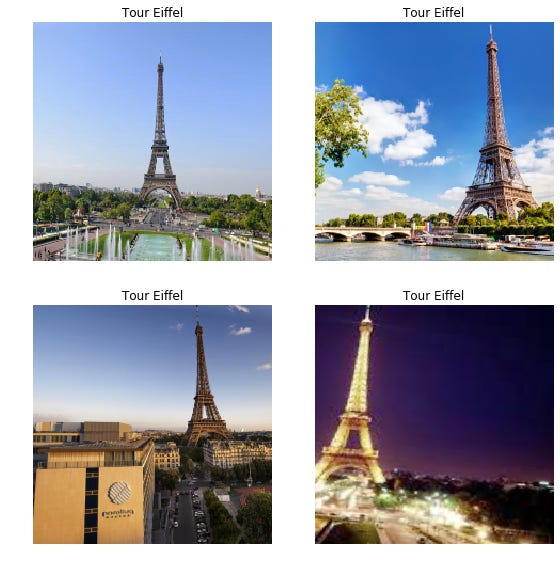Display a batch of 4 images generated by the ImageDataBunch after applying the transformations defined in get_transforms ()